Questions about these zeroth law variations?
Know of other zeroth law definitions?
TOP
P.S.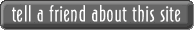Inception: 11/16/05
Variations of the 0th Law of ThermodynamicsMaxwell, J. (1872). Source: Perrot, P. (1998). A to Z of Thermodynamics (dictionary). New York: Oxford University Press.

 Fowler, R. (1931). Source: [www.jcphysics.com] [URL] + Cengel, Y. & Boles, M. (2002). Thermodynamics – an Engineering Approach, 4th Ed. (textbook). New York: McGraw Hill.

 Bent, H. (1965). The Second Law – an Introduction to Classical and Statistical Thermodynamics.  (textbook). New York: Oxford University Press.

 Adkins, C. (1983). Equilibrium Thermodynamics, 3rd Ed. (textbook). Cambridge: Cambridge University Press.

 Black, W. & Hartley, J. (1996). Thermodynamics, 3rd Ed. (textbook). New York: Harper Collins.

 Kondepudi, D. & Prigogine, I. (1998). Modern Thermodynamics – From Heat Engines to Dissipative Structures (textbook). New York: John Wiley & Sons.

 Wark, R. & Richards, D. (1999). Thermodynamics, 6th Ed. (textbook). New York: McGraw-Hill.

 Schroeder, D. (2000). An Introduction to Thermal Physics. (textbook). New York: Addison Wesley Longman.

 Exell, R.H.B. (2001). "The Zeroth Law of Thermodynamics" [URL]. King Mongkut's University of Technology Thonburi.

[10-11] Cengel, Y. & Boles, M. (2002). Thermodynamics – an Engineering Approach, 4th Ed. (textbook). New York: McGraw Hill.

 Schneider, E. & Sagan, D. (2005). Into The Cool - Energy Flow, Thermodynamics, and Life. Chicago: University of Chicago Press.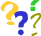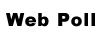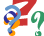Pick the best 0th Law:#1#2#3#4#5#6#7#8#9#10#11#12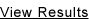POLL:
of the 0th LawCONTENTS

1-10; 1906 - 2002
11-20; 2002 - Present

Poll
Notes
Sources
Guest Book
AnchorsRalph Fowler [1889-1944]
This list is under-construction (11/24/05)SIGNOUT
 1 Two systems A and B in thermal equilibrium with a third system C are in thermal equilibrium with one another. Maxwell

 2 The (heat transfer) principle of Fowler. Fowler

 3 If bodies A and B are found to be in thermal equilibrium with each other: (ΔE/ΔS)A = (ΔE/ΔS)Band bodies A and C are found to be in thermal equilibrium with each other: (ΔE/ΔS)A = (ΔE/ΔS)C it follows that: (ΔE/ΔS)B = (ΔE/ΔS)C implying that bodies B and C when tested against each other would be found to be in thermal equilibrium. Bent

 4 If two systems are separately in thermal equilibrium with a third, then they must also be in thermal equilibrium with each other. Adkins

 5 When two bodies have equality of temperature with a third body, they have equality of temperature with each other. Black & Hartley

 6 The “transitivity” of the state of equilibrium, where two or more systems that interact and exchange energy and / or matter will eventually reach the state of thermal equilibrium in which the temperatures of all systems are equal. Kondepudi & Prigogine

 7 When each of two systems is in thermal equilibrium with a third system, they also will be in thermal equilibrium with each other. Wark & Richards

 8 If system A is in thermal equilibrium with system B, and system B is in thermal equilibrium with system C, then system A is in thermal equilibrium with system C. Schroeder

 9 Two thermodynamic systems in thermal equilibrium with the same environment are in thermal equilibrium with each other. Exell

 10 If two bodies are in thermal equilibrium with a third body, they are also in thermal equilibrium with each other. Cengel & Boles

 11 Two bodies are in thermal equilibrium if both have the same temperature reading even if they are not in contact. Cengel & Boles

 12 When a closed thermodynamic system within rigid heat-proof walls reaches the point of no more change, the system has reached a state of thermal equilibrium. Schneider & SaganOth Law PollOth Law NotesOth Law SourcesJames Maxwell [1831-1879]Institute of Human Thermodynamics